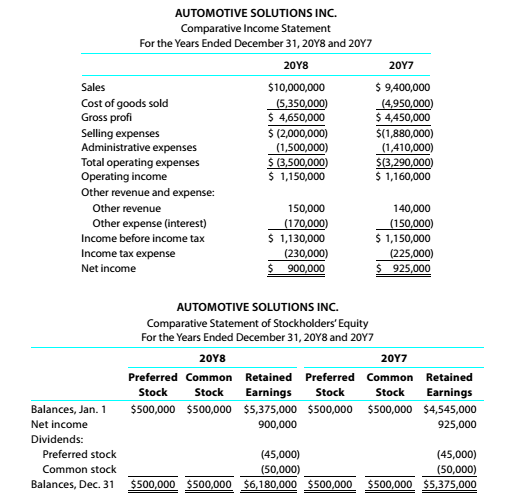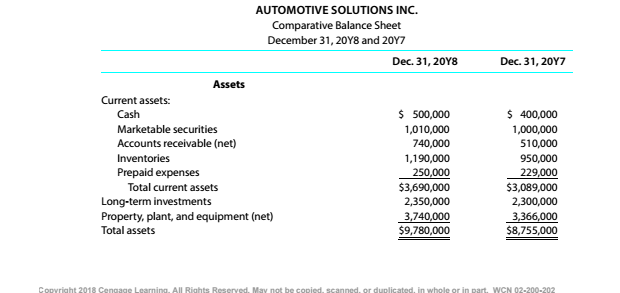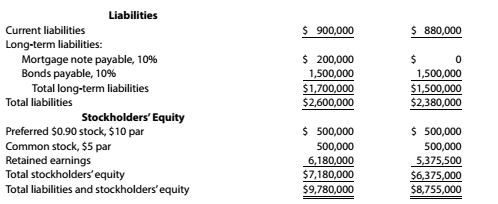Chapter 9, Problem 9.4.1P

Chapter
Section
Textbook Problem

Twenty metrics of liquidity, solvency, and profitabilityThe comparative financial statements of Automotive Solutions Inc. are as follows. The market price of Automotive Solutions Inc. common stock was $119.70 on December 31, 20Y8InstructionsWorking To determine Concept Introduction: Current Ratio: Current Ratio is the measure of the company's ability to pay off its current liabilities using its current assets. It is calculated by dividing the total current assets by total current liabilities. The formula of the current ratio is as follows: Current Ratio=Current assetsCurrent liabilities To Calculate: The working capital. Explanation The working capital is calculated as follows:  20Y8 20Y7 Total Current Assets (A)$ 3,690,000 \$ ...

Still sussing out bartleby?

Check out a sample textbook solution.

See a sample solution

The Solution to Your Study Problems

Bartleby provides explanations to thousands of textbook problems written by our experts, many with advanced degrees!

Get Started

Why should policymakers think about incentives?

Essentials of Economics (MindTap Course List)

Define the term marketing

MKTG 12:STUDENT ED.-TEXT

Financial ratio analysis is conducted by three main groups of analysts: credit analysts, stock analysts, and ma...

Fundamentals of Financial Management, Concise Edition (with Thomson ONE - Business School Edition, 1 term (6 months) Printed Access Card) (MindTap Course List)